## Analogy

#### Analogy

Direction: Each of the following questions consists of two sets of figures. Figures (a), (b), (c) and (d) constitute the Question Set while figures (1), (2), (3), (4) and (5) constitute the Answer Set. There is a definite relationship between figures (a) and (b). Figure (a) and (b) are related in a particular way or manner. Establish the same relationship between (c) and (d).

1. Select a suitable figure from the answer figures that would replace the question mark (?) from question figure.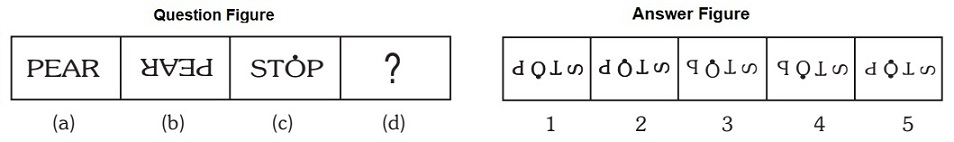1. The complete figure rotates through 180 degree and the last element on the right side rotates 90 degree.

##### Correct Option: A

The complete figure rotates through 180 degree and the last element on the right side rotates 90 degree.

1. Select a suitable figure from the Answer Figures that would replace the question mark (?) from question figure.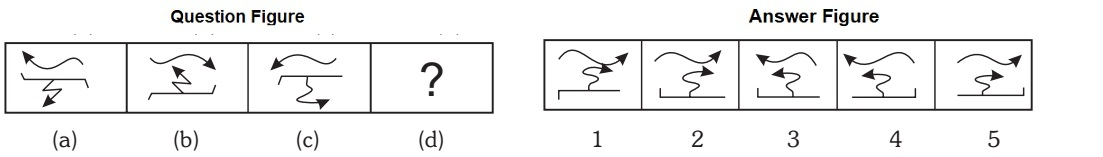1. The upper element moves 180 degree its head inverted and the lower one vertically inverted.

##### Correct Option: B

The upper element moves 180 degree its head inverted and the lower one vertically inverted.

1. Select a suitable figure from the Answer Figures that would replace the question mark (?) from question figure.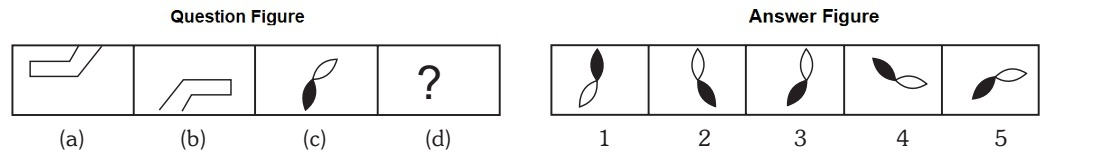1. The figure rotates through 180 degree.

##### Correct Option: C

The figure rotates through 180 degree.

1. Select a suitable figure from the Answer Figures that would replace the question mark (?) from question figure.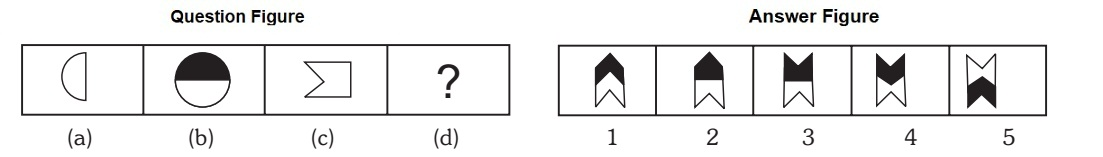1. The figure rotates 90 degree anti –clock wise and shaded inverted image added to the top.

##### Correct Option: C

The figure rotates 90 degree anti –clock wise and shaded inverted image added to the top.

1. Select a suitable figure from the answer figures that would replace the question mark (?) from question figure.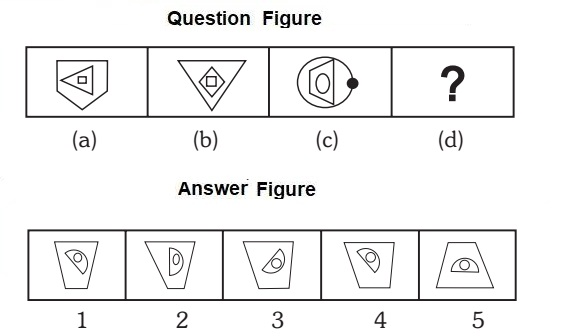1. The outer most element reduces its side and inverted. The middle element moves 90 degree anti – clock wise and moves to outer side and the inner one moves to middle position at 45 degree anti clock wise.

##### Correct Option: A

The outer most element reduces its side and inverted. The middle element moves 90 degree anti – clock wise and moves to outer side and the inner one moves to middle position at 45 degree anti clock wise.